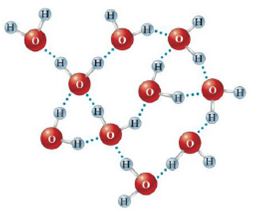Chapter 10, Problem 15Q

Chapter
Section
Textbook Problem

# In the diagram below, which lines represent the hydrogen bonding?a. the dotted lines between the hydrogen atoms of one water molecule and the oxygen atoms of a different water moleculeb. the solid lines between a hydrogen atom and oxygen atom in the same water moleculec. Both the solid lines and dotted lines represent hydrogen bonding.d. There are no hydrogen bonds represented in the diagram.

Interpretation Introduction

Interpretation:

The hydrogen bonding in given diagram should be explained.

Concept Introduction:

Phase diagram:

• The plot of temperature against pressure is called phase diagram. It contains the information of boiling and melting points and critical temperature and pressure of the substance.
Explanation

Explanation

Record the given data:

Fig...

### Still sussing out bartleby?

Check out a sample textbook solution.

See a sample solution

#### The Solution to Your Study Problems

Bartleby provides explanations to thousands of textbook problems written by our experts, many with advanced degrees!

Get Started

## Additional Science Solutions

#### Find more solutions based on key concepts